# 深度学习与计算机视觉教程(16) | 生成模型(PixelRNN,PixelCNN,VAE,GAN)（CV通关指南·完结🎉）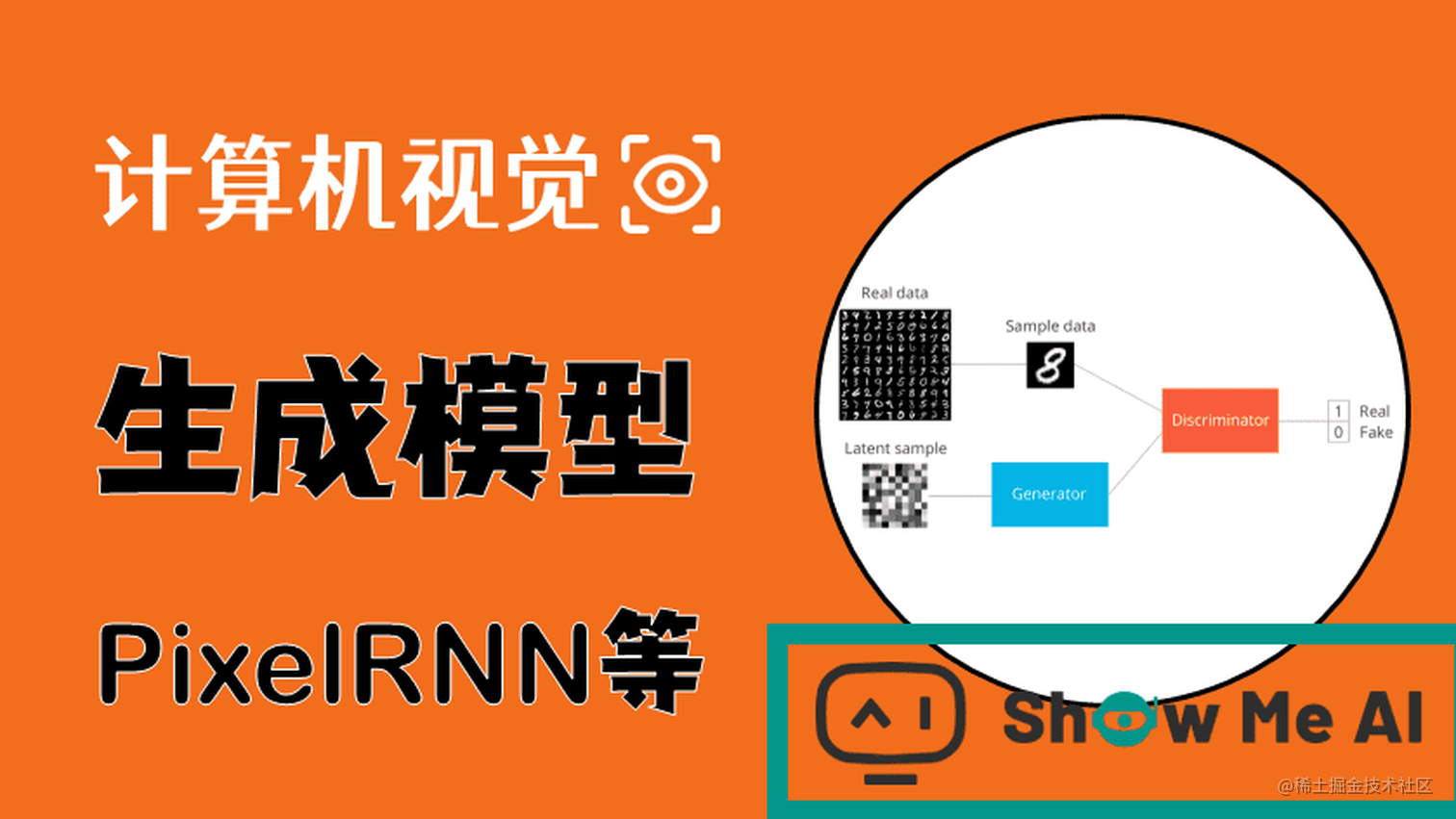# 引言

CS231n第13讲给大家介绍的是无监督学习（Unsupervised Learning）以及生成模型的一些知识。

## 本篇重点

• 无监督学习
• 生成模型
• Pixel RNN/CNN
• 变分自编码器（VAE）
• 生成对抗网络（GAN）

# 1.无监督学习

## 1.1 聚类（k-Means）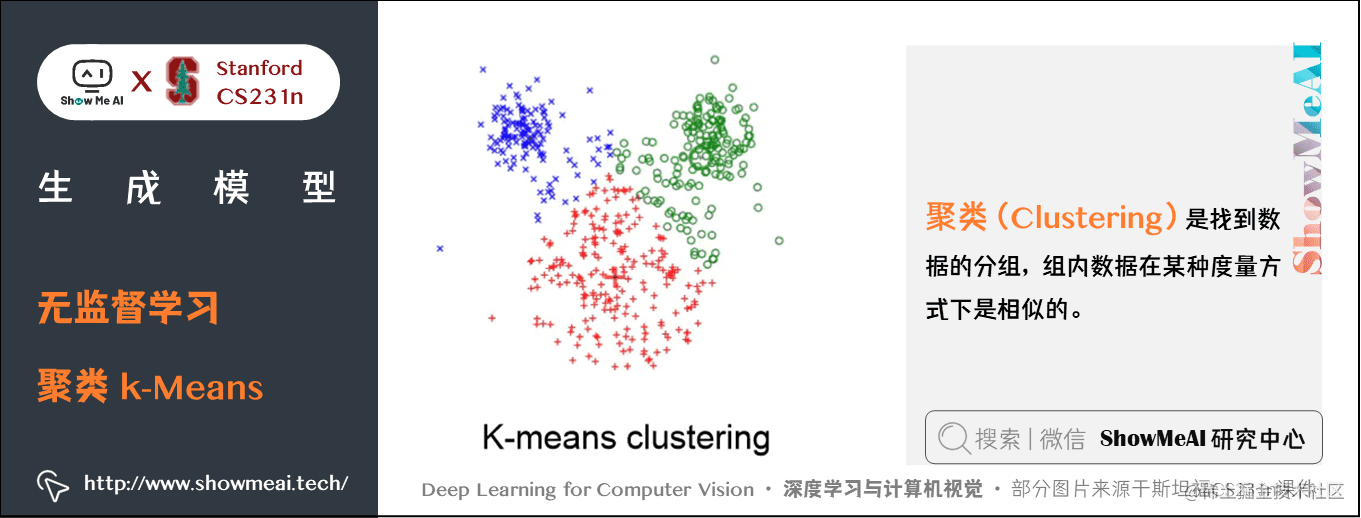## 1.2 PCA（主成分分析）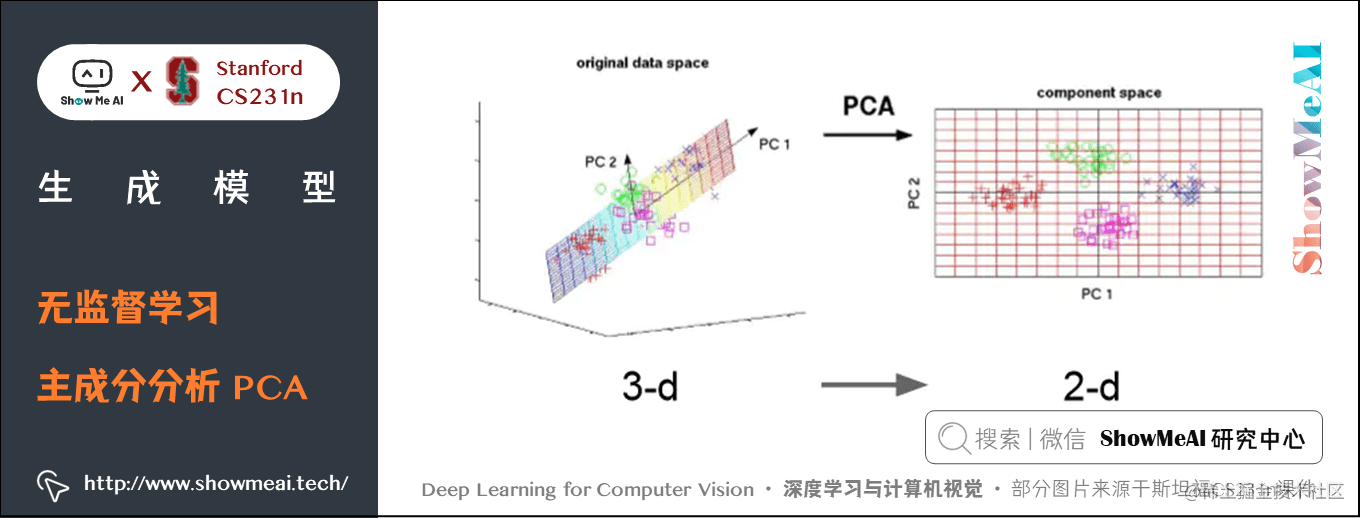## 1.3 特征学习（Feature Learning）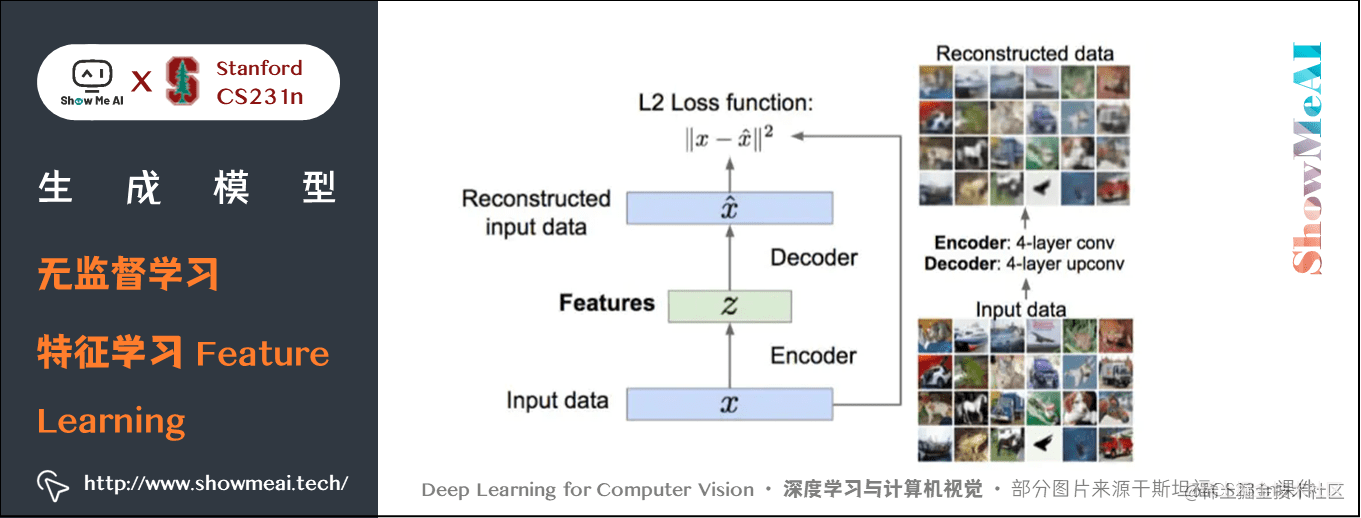## 1.4 密度估计（ Density Estimation）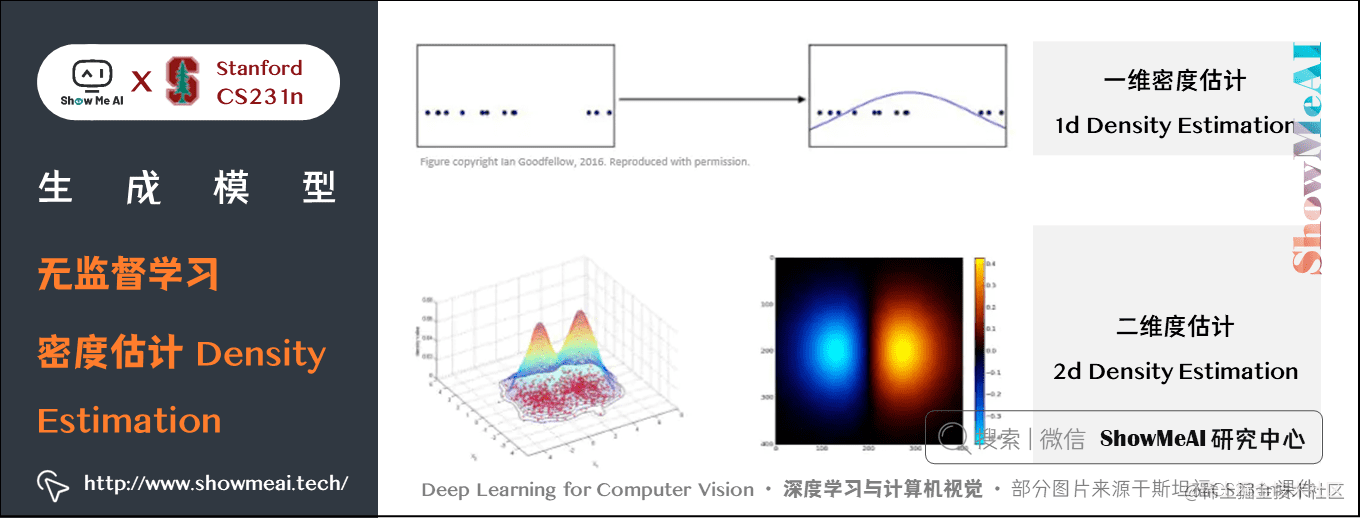# 2.生成模型（Generative Models）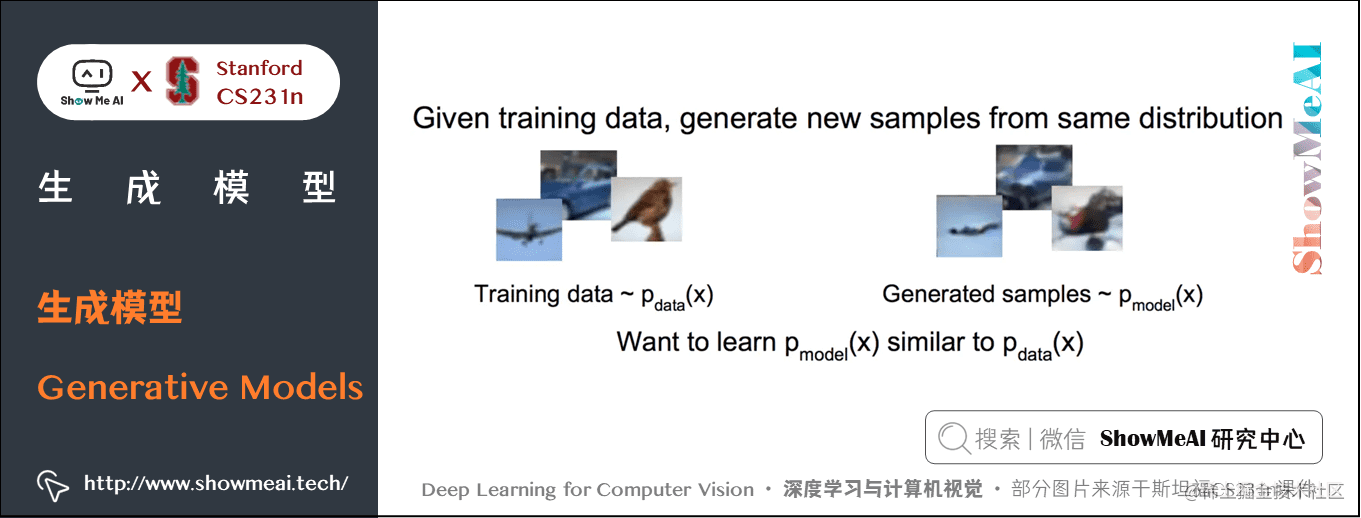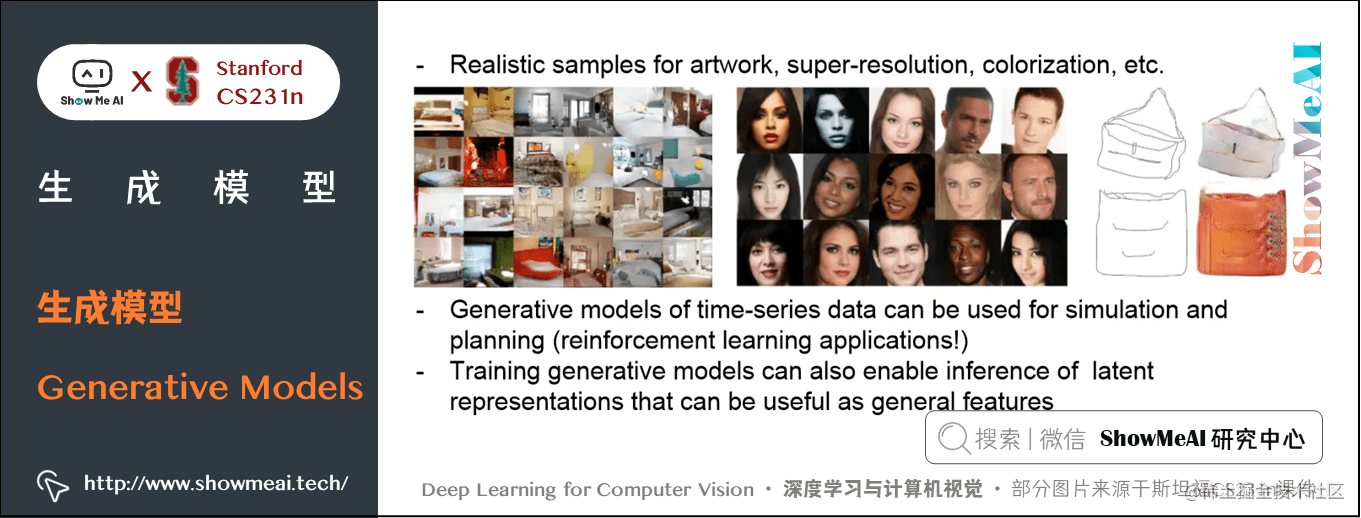## 2.1 PixelRNN 和 PixelCNN

PixelRNN 和 PixelCNN 使用概率链式法则来计算一张图片出现的概率。其中每一项为给定前 $i-1$ 个像素点后第 $i$ 个像素点的条件概率分布。这个分布通过神经网络 RNN 或 CNN 来建模，再通过最大化图片 $x$ 的似然概率来学习出 RNN 或 CNN 的参数。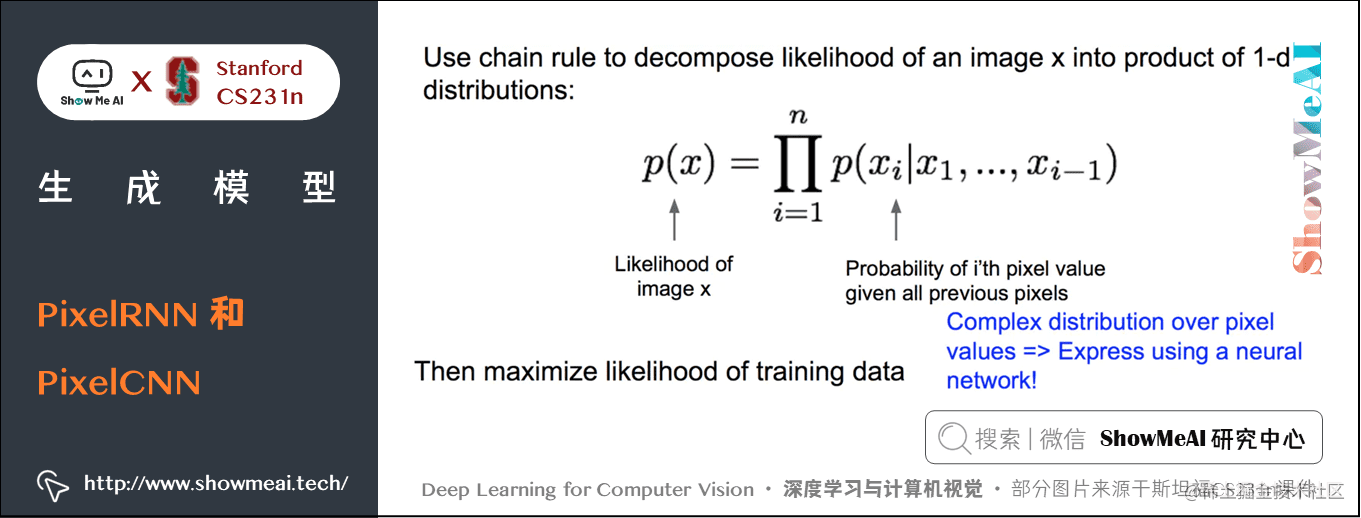$p(x)=\prod_{i=1}^{n} p(x_{i} \mid x_{1}, \ldots, x_{i-1})$

• $p(x)$: 图像x的似然概率
• $p(x_{i} \mid x_{1}, \ldots, x_{i-1})$: 条件概率

PixelRNN 中，从左上角开始定义「之前的像素」。由于 RNN 每个时间步的输出概率都依赖于之前所有输入，因此能够用来表示上面的条件概率分布。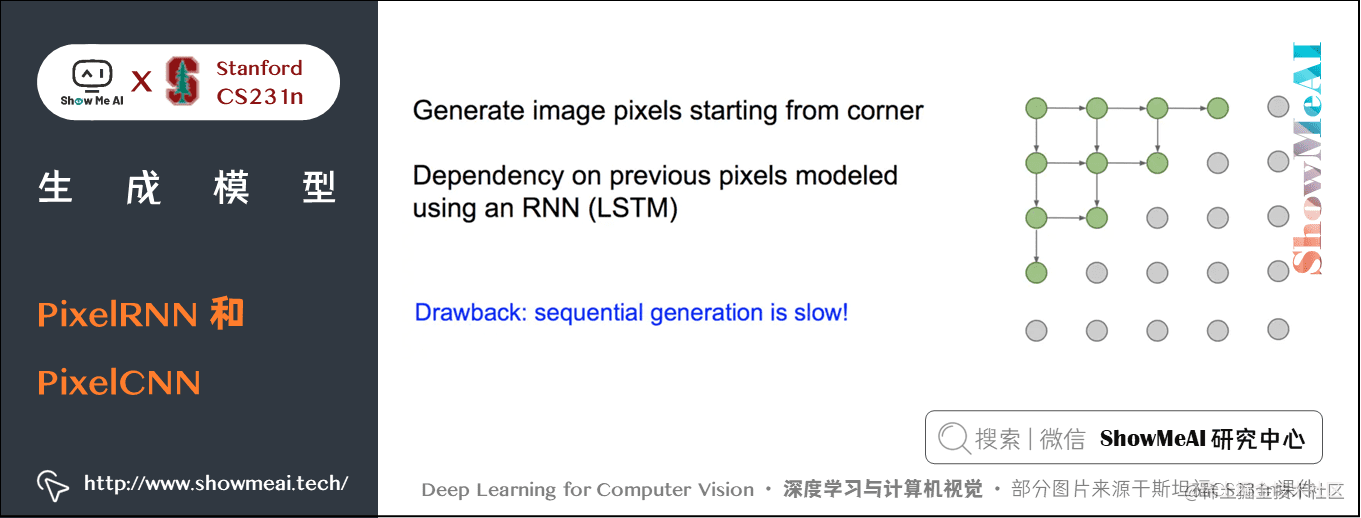PixelCNN中，使用一个CNN来接收之前的所有像素，并预测下一个像素的出现概率：

$p(x)=\prod_{i=1}^{n} p(x_{i} \mid x_{1}, \ldots, x_{i-1})$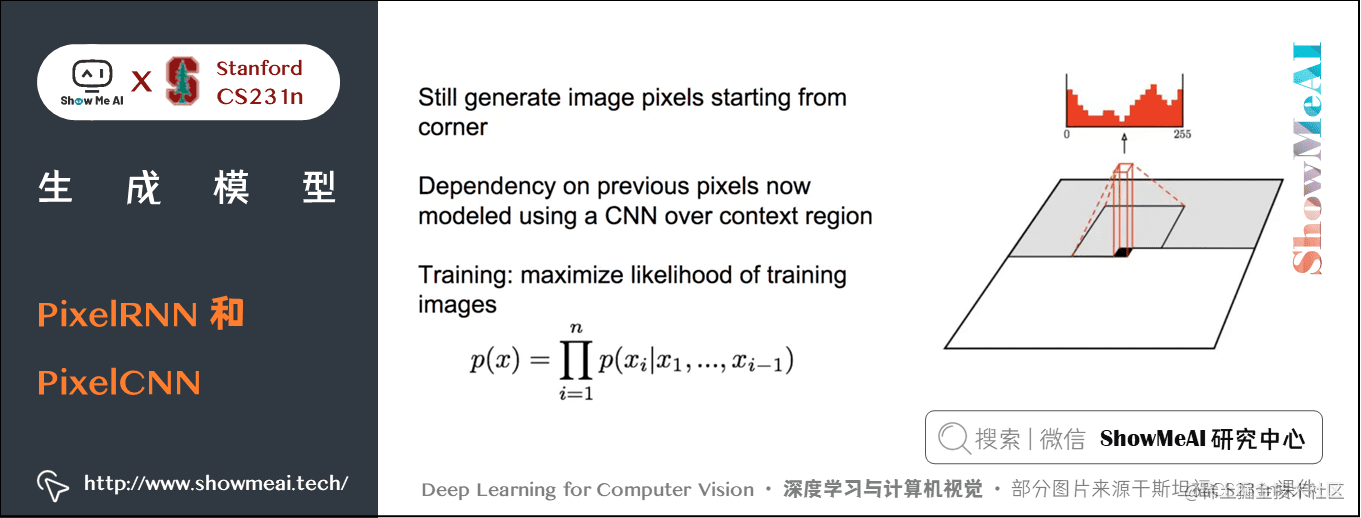PixelRNN 和 PixelCNN 能显式地计算似然 $p(x)$，是一种可优化的显式密度模型，该方法给出了一个很好的评估度量，可以通过计算的数据的似然来度量出生成样本有多好。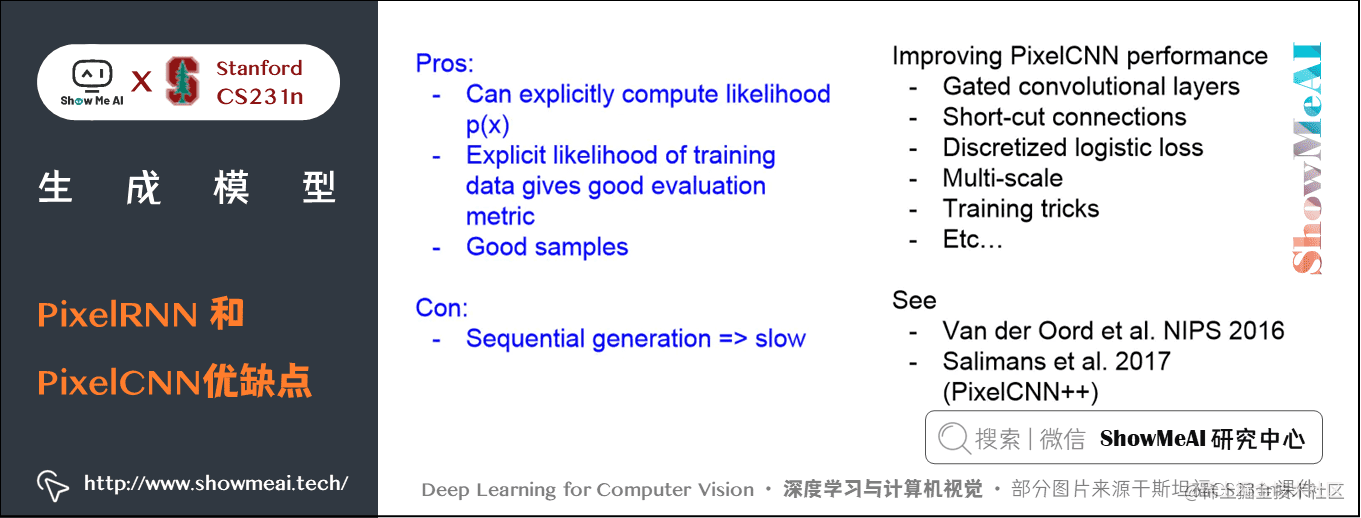## 2.2 变分自编码器（VAE）

PixelCNN定义了一个易于处理的密度函数，我们可以直接优化训练数据的似然；而我们下面介绍到的变分自编码器方法中，密度函数就不易处理了，我们要通过附加的隐变量 $z$ 对密度函数进行建模：

$p_{\theta}(x)=\int p_{\theta}(z) p_{\theta}(x \mid z) d z$

### 1) 自编码器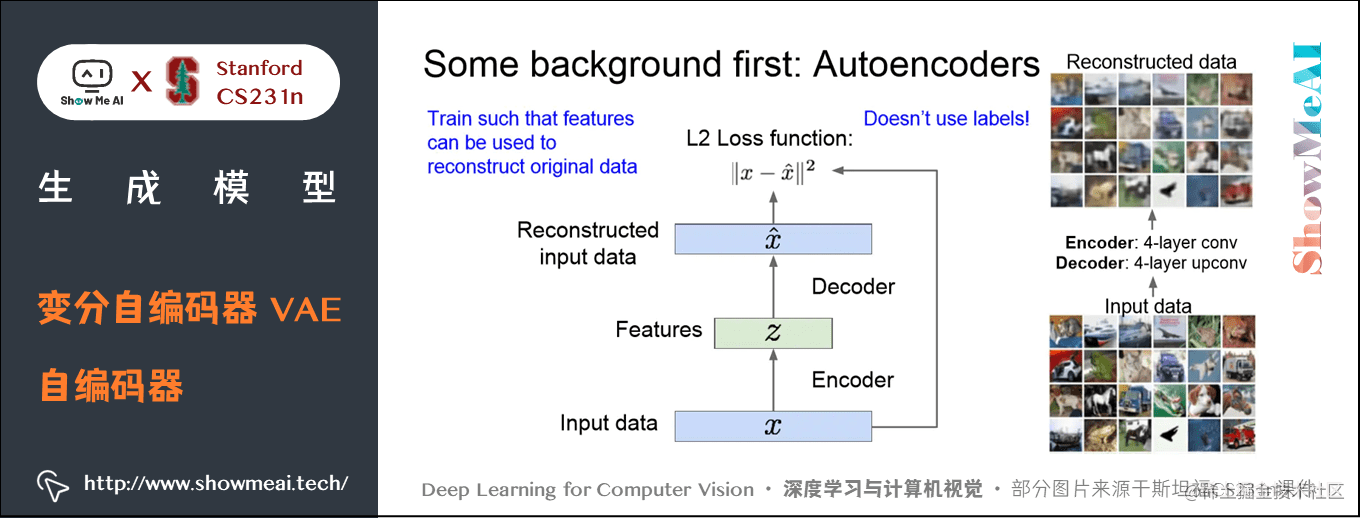### 解码器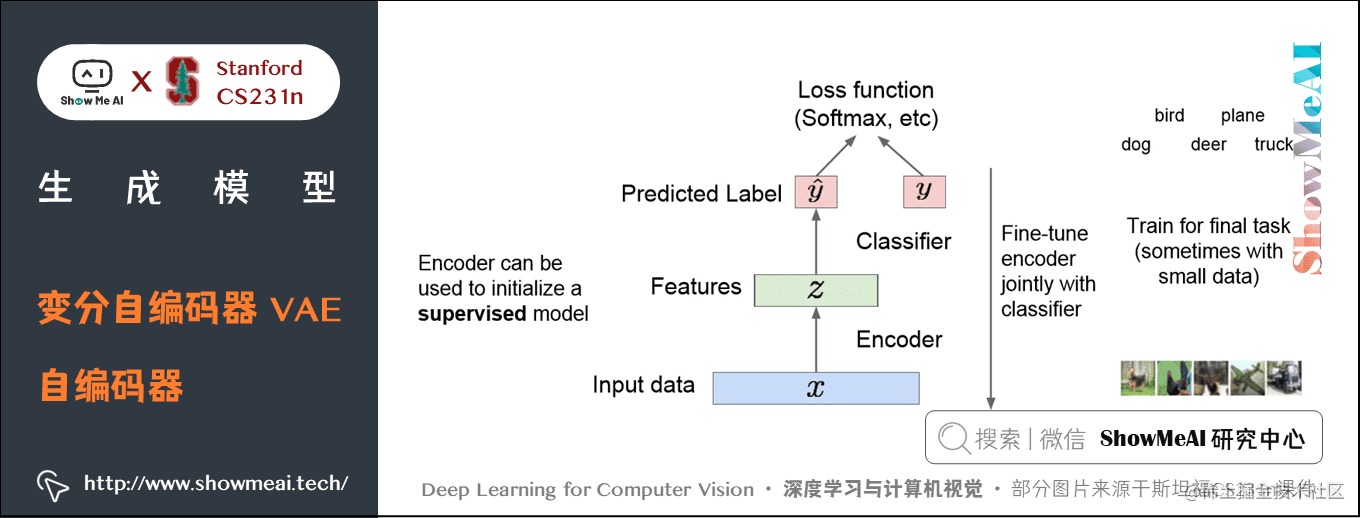### 2) VAE的思想

VAE模型的思路是，如果我们无法直接获得样本 $x$ 的分布，那么我们可以假设存在一个 $x$ 对应的隐式表征 $z$$z$ 的分布是一个先验分布（比如高斯分布或其他简单的分布）。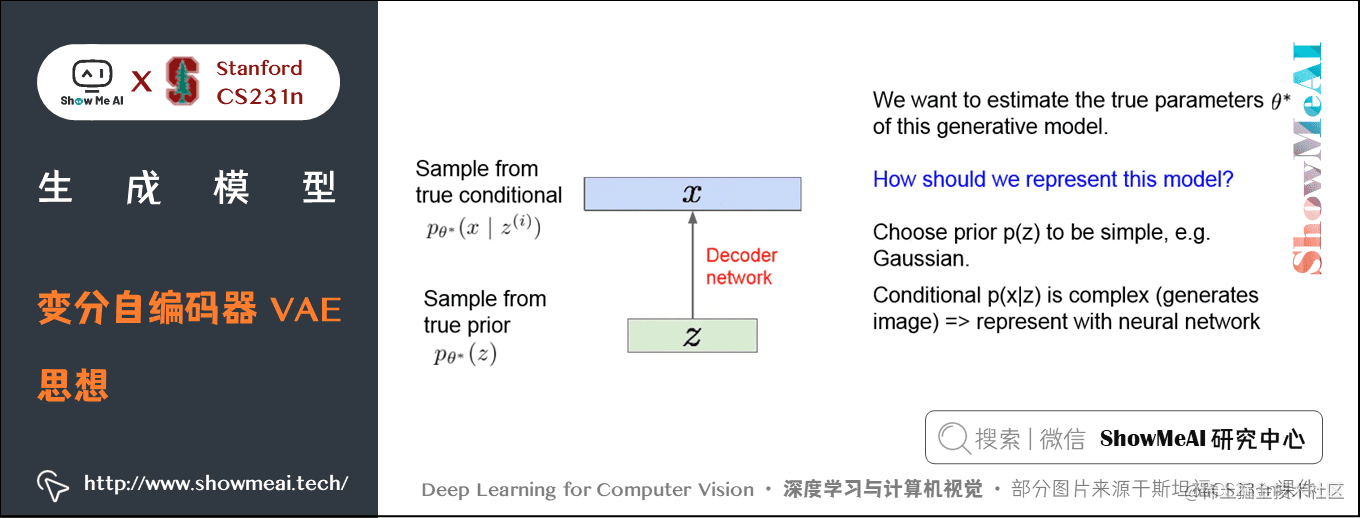### 3) 如何训练VAE

$p_{\theta}(x)=\int p_{\theta}(z) p_{\theta}(x \mid z) d z$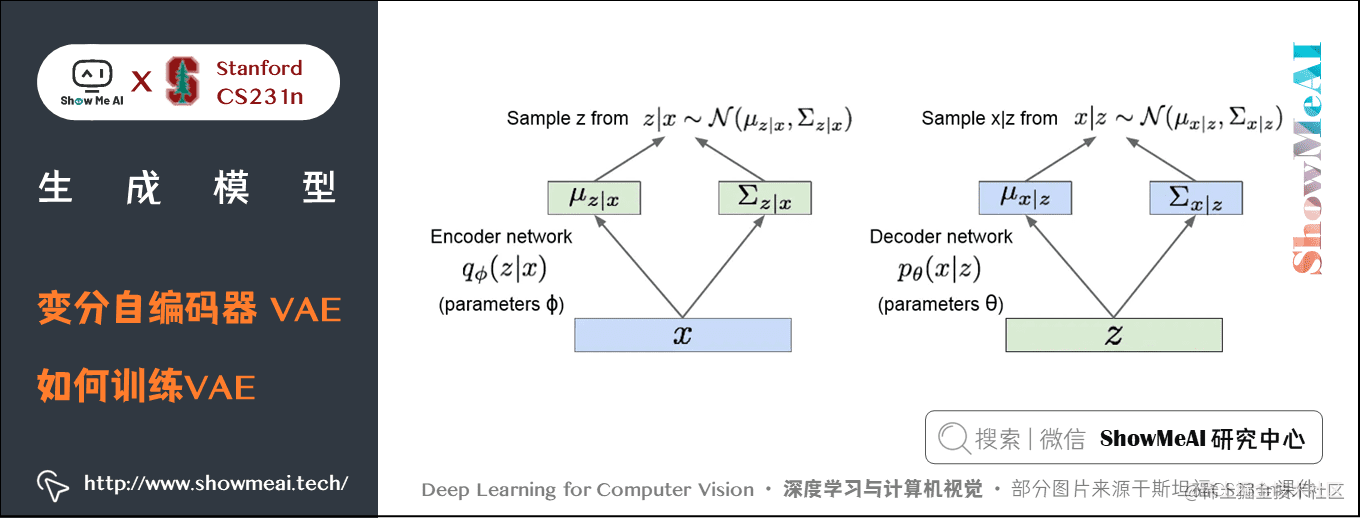$\log \mathrm{L}=\log p(x)=\log p(x) \cdot 1=\log p(x) \int_{z} q(z \mid x) d z$

\begin{aligned} \log \mathrm{L} &=\log p(x) \int_{z} q(z \mid x) d z \\ &=\int_{z} q(z \mid x) \log \frac{p(x, z)}{p(z \mid x)} d z \\ &=\int_{z} q(z \mid x)\left[\log \frac{p(x, z)}{q(z \mid x)}-\log \frac{p(z \mid x)}{q(z \mid x)}\right] d z \\ &=\int_{z} q(z \mid x) \log \frac{p(x, z)}{q(z \mid x)} d z+\int_{z} q(z \mid x) \log \frac{q(z \mid x)}{p(z \mid x)} d z \\ &=\int_{z} q(z \mid x) \log \frac{p(x, z)}{q(z \mid x)} d z+D_{K L}(q(z \mid x) \| p(z \mid x)) \end{aligned}

\begin{aligned} \int_{z} q(z \mid x) \log \frac{p(x, z)}{q(z \mid x)} d z &=\int_{z} q(z \mid x) \log p(x \mid z) d z+\int_{z} q(z \mid x) \log \frac{p(x)}{q(z \mid x)} d z \\ &=\mathrm{E}_{z \sim q}[\log p(x \mid z)]-D_{K L}(q(z \mid x) \| p(z)) \end{aligned}

$\log p(x)=\mathrm{E}_{z \sim q}[\log p(x \mid z)]-D_{K L}(q(z \mid x) \| p(z))+D_{K L}(q(z \mid x) \| p(z \mid x))$

$p_{\theta}(z \mid x)=p_{\theta}(x \mid z) p_{\theta}(z) / p_{\theta}(x)$
$p_{\theta}(x)=\int p_{\theta}(z) p_{\theta}(x \mid z) d z$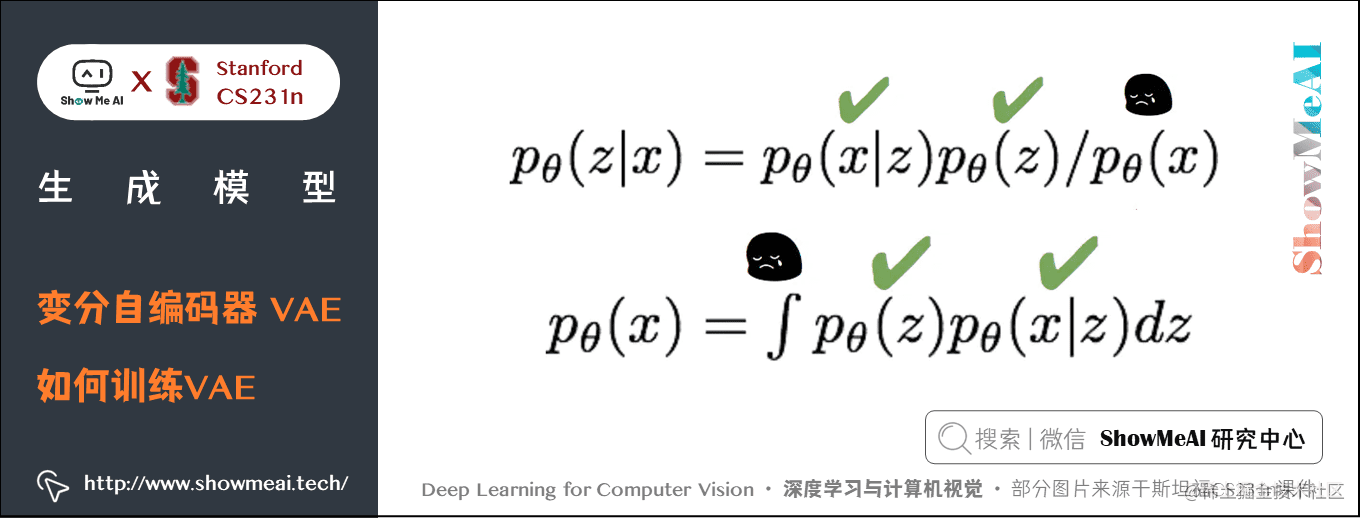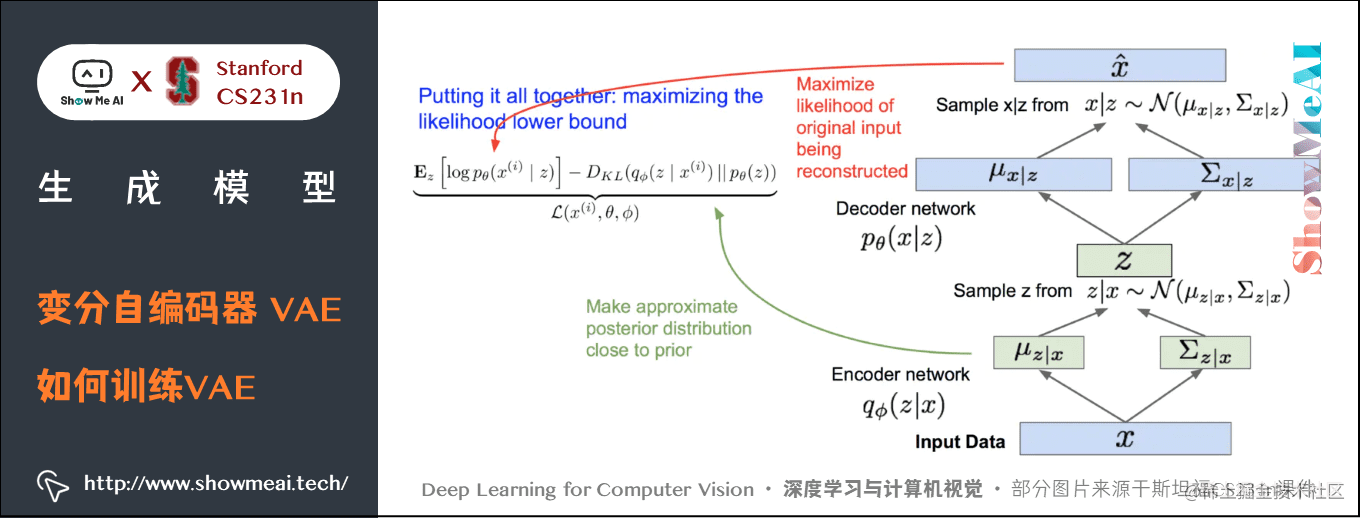① 第1项是对所有采样的 $z$ 取期望， $z$$x$ 经过编码器网络采样得到，对 $z$ 采样然后再求所有 $z$ 对应的 $p(x \mid z)$ 。让 $p(x \mid z)$ 变大，就是最大限度地重构数据。

② 第2项是让KL的散度变小，让我们的近似后验分布和先验分布变得相似，意味着我们想让隐变量z遵循我们期望的分布类型。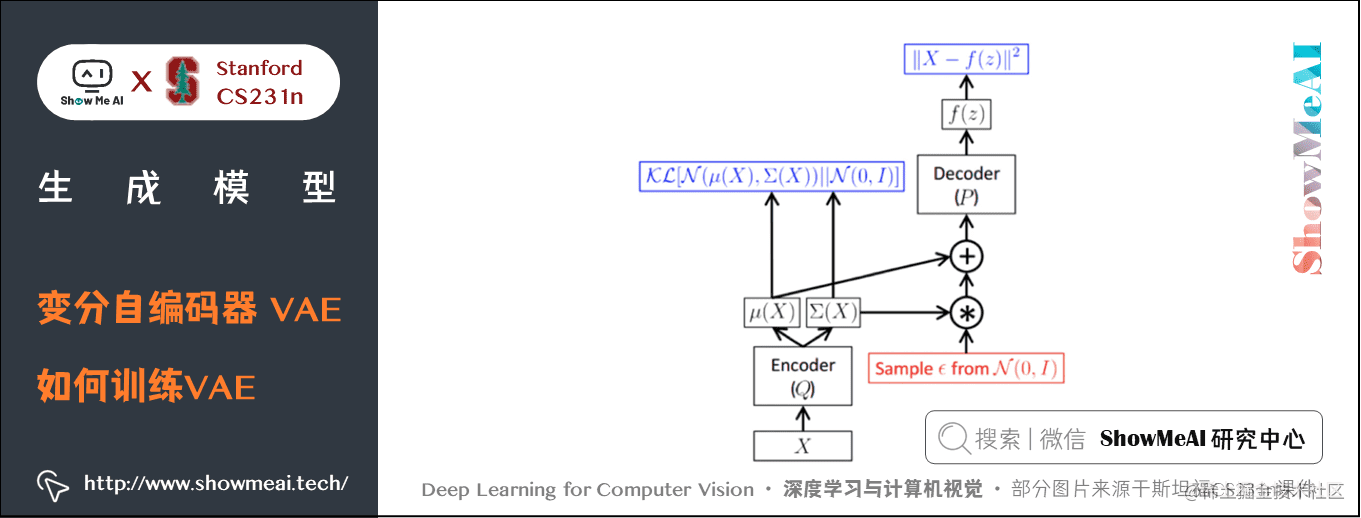### 4) VAE的优缺点

VAEs优点

VAEs 就生成式模型来说是一种有据可循的方法，它使得查询推断称为可能，如此一来便能够推断出像 $q(z \mid x)$ 这样的分布，这些东西对其他任务来说会是很有用的特征表征。

VAEs缺点

## 2.3 生成对抗网络(Generative Adversarial Nets, GAN)

### 1) GAN的核心思路

• 判别器负责辨别哪些样本是生成器生成的假样本，哪些是从真实训练集中抽出来的真样本。
• 生成器负责利用随机噪声 $z$ 生成假样本，它的职责是生成尽可能真的样本以骗过判别器。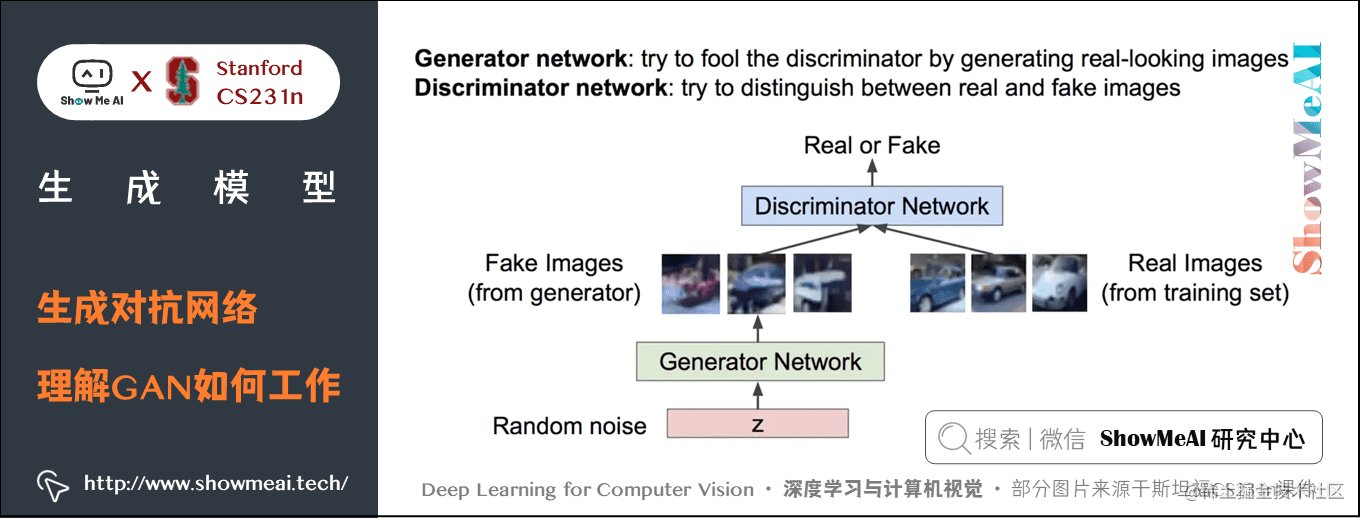$\min _{\theta_{g}} \max _{\theta_{d}}\left[\mathbb{E}_{x \sim p_{\text {data }}} \log D_{\theta_{d}}(x)+\mathbb{E}_{z \sim p(z)} \log \left(1-D_{\theta_{d}}\left(G_{\theta_{g}}(z)\right)\right)\right]$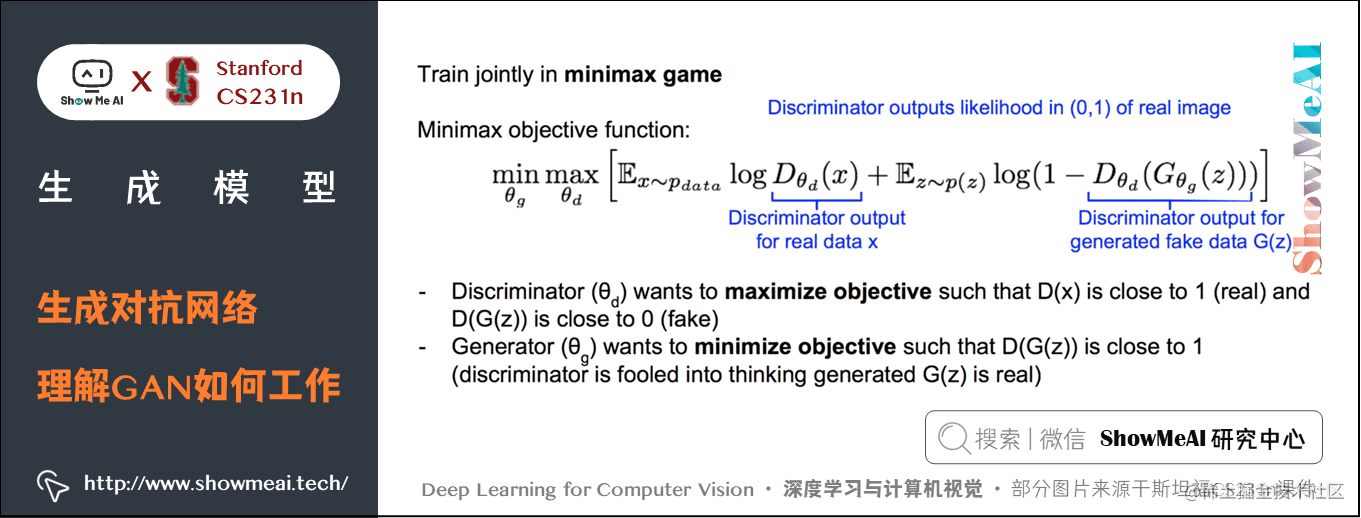• 让目标函数在 $\theta_ g$ 上取得最小值，同时要在 $\theta_ d$ 上取得最大值。
• 其中：$\theta_g$ 是生成器网络g的参数，$\theta_d$指的是判别器网络的参数。

• 第1项是在训练数据的分布上 $log(D(x))$ 的期望，$log(D(x))$ 是判别器网络在输入为真实数据（训练数据）时的输出，该输出是真实数据从分布 p-data 中采样的似然概率；
• 第2项是对 $z$ 取期望， $z$ 是从 $p(z)$ 中采样获得的，这意味着从生成器网络中采样，同时 $D(G(z))$ 这一项代表了以生成的伪数据为输入判别器网路的输出，也就是判别器网络对于生成网络生成的数据给出的判定结果。

### 训练方法

① 对判别器进行梯度上升，学习到 $\theta_d$ 来最大化该目标函数；

$\max _{\theta_{d}}\left[\mathbb{E}_{x \sim p_{\text {data }}} \log D_{\theta_{d}}(x)+\mathbb{E}_{z \sim p(z)} \log \left(1-D_{\theta_{d}}\left(G_{\theta_{g}}(z)\right)\right)\right]$

② 对生成器进行梯度下降， $\theta_g$ 进行梯度下降最小化目标函数（此时目标函数如下的部分，因为只有它与 $\theta_g$ 有关）

$\min _{\theta_{g}} \mathbb{E}_{z \sim p(z)} \log \left(1-D_{\theta_{d}}\left(G_{\theta_{g}}(z)\right)\right)$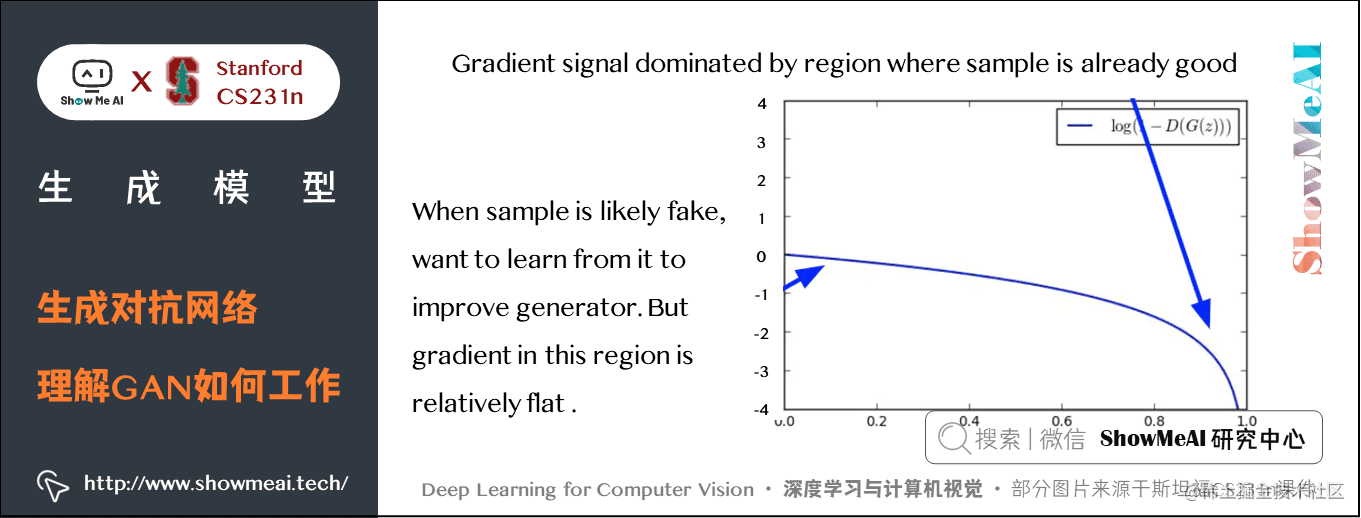$\max _{\theta_{g}} \mathbb{E}_{z \sim p(z)} \log \left(D_{\theta_{d}}\left(G_{\theta_{g}}(z)\right)\right)$

• 训练判别器

• 对于判别器网络的k个训练步，先从噪声先验分布 $z$ 中采样得到一个小批量样本，接着从训练数据 $x$ 中采样获得小批量的真实样本，下面要做的将噪声样本传给生成器网络，并在生成器的输出端获得生成的图像。
• 此时我们有了一个小批量伪造图像和小批量真实图像，我们有这些小批量数据在判别器生进行一次梯度计算，接下来利用梯度信息更新判别器参数，按照以上步骤迭代几次来训练判别器。

• 训练生成器

• 在这一步采样获得一个小批量噪声样本，将它传入生成器，对生成器进行反向传播，来优化目标函数。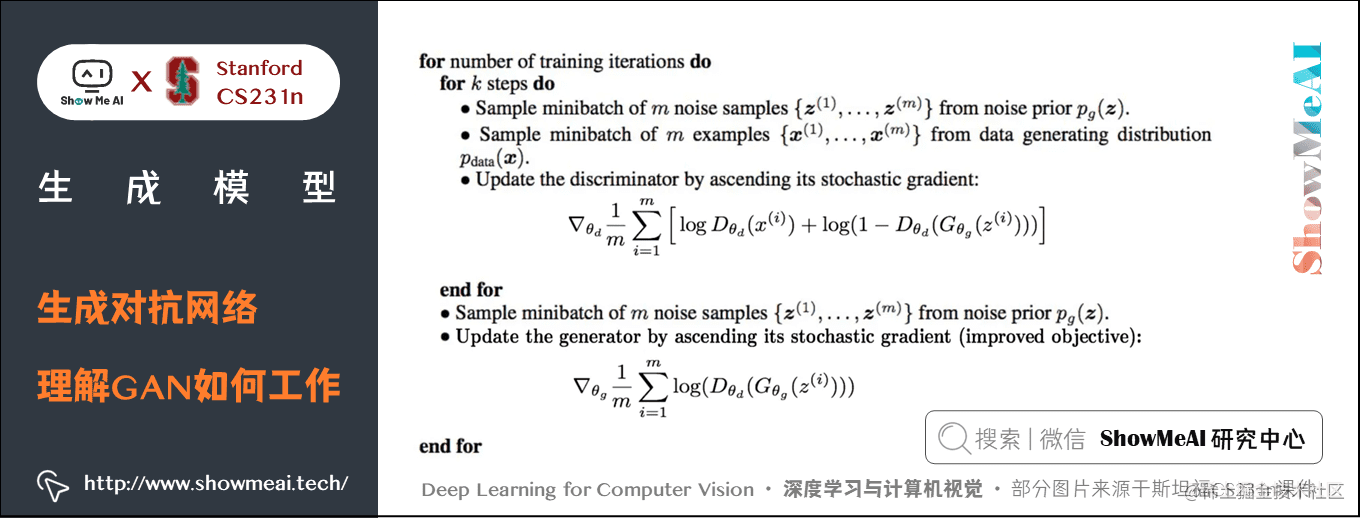### 2) GAN的探索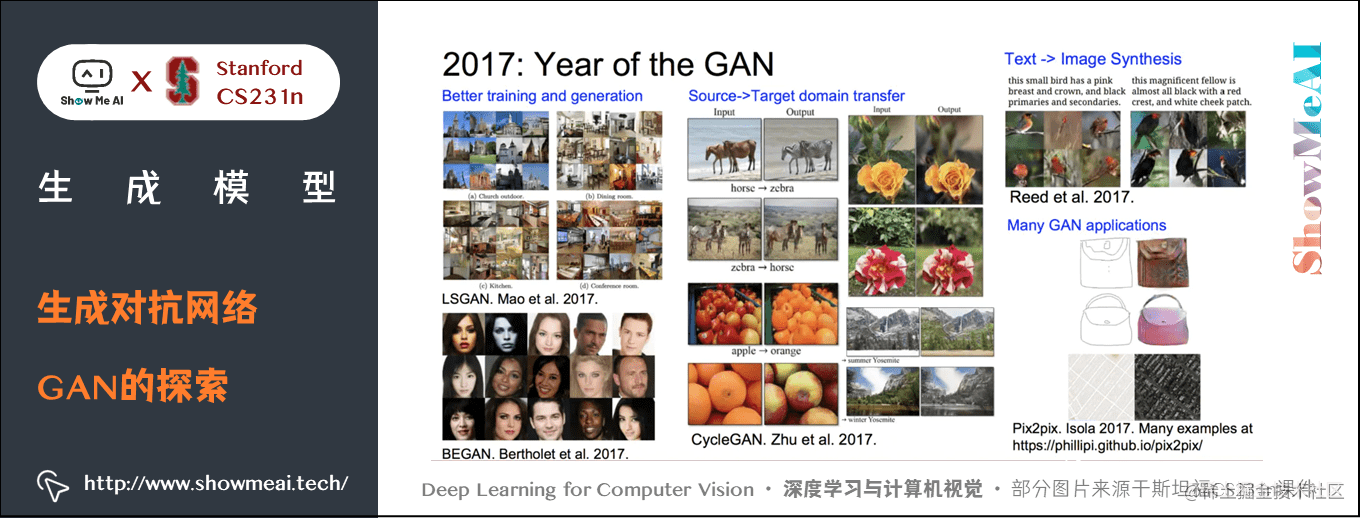• 传统的GAN生成的样本还不是很好，这篇论文在GAN中使用了CNN架构，取得了惊艳的生成效果：[Radford et al, “Unsupervised Representation Learning with Deep Convolutional Generative Adversarial Networks”, ICLR 2016]
• Wasserstein GAN 一定程度解决了GAN训练中两个网络如何平衡的问题。
• 用GAN来做text -> image

### GAN 的优点

• GAN通过一种博弈的方法来训练，通过两个玩家的博弈从训练数据的分布中学会生成数据。
• GAN可以生成目前最好的样本，还可以做很多其他的事情。

### GAN 的缺点

• GAN没有显式的密度函数（它是利用样本来隐式表达该函数）
• GAN不好训练且不稳定，我们并不是直接优化目标函数，我们要努力地平衡两个网络。

### GAN 的热门研究方法

• 更好的损失函数设计，更稳定的训练方式（例如Wasserstein GAN, LSGAN及其他）
• 条件GAN，GAN的各种应用领域探索

# 4.要点总结

• PixelCNN 和 PixelRNN 他们是显式密度模型，该模型优化的是一个显式的似然函数并产生良好的样本，但是效率很低，它是一个顺序的生成过程。
• VAE 优化的是一个似然函数的下界，它会产生一个有用的隐式表征，可以用它来进行查询推断，生成的样本也不是特别好。
• GAN 是目前能生成最好样本的模型，但是训练需要技巧且不稳定，查询推断上也有一些问题。
• 还有一些将模型的优点结合起来做的研究。

# ShowMeAI 系列教程推荐•••• ShowMeAI
1年前•• NingBo
28天前